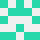# 如何在lua中添加技能点限制?

``````  function lvl()
if xp >= xpr then
level = level + 1
xpr = xpr * 1.5
xp = 0
io.write("祝贺你!你没有死亡并失去一切!", "\n")
io.write("你的等级现在是:" .. level .. "\n")
sp = sp + 6
io.write("你想分配你的" .. sp .. "技能点吗?", "\n")
if sac == "是" then
io.write("你想给力量设多少技能点?", "\n")
if sp == 0 then
io.write("你没有任何技能点!", "\n")
else
str = str + strsp
io.write("力量: ", str, "\n")
end
io.write("你想给灵巧设多少技能点?", "\n")
if sp == 0 then
io.write("你没有任何技能点!", "\n")
else
dex = dex + dexsp
io.write("灵巧: ", dex, "\n")
end
io.write("你想给体质设多少技能点?", "\n")
if sp == 0 then
io.write("你没有任何技能点!", "\n")
else
con = con + consp
io.write("体质: ", str, "\n")
end
io.write("你想给智力设多少技能点?", "\n")
if sp <= 0 then
io.write("你没有任何技能点!", "\n")
else
int = int + intsp
end
io.write("你想给智慧设多少技能点?", "\n")
if sp <= 0 then
io.write("你没有任何技能点!", "\n")
else
wis = wis + wissp
end
io.write("你想给魅力设多少技能点?", "\n")
if sp <= 0 then
io.write("你没有任何技能点!", "\n")
else
cha = cha + chasp
end

elseif xp < xpr then
io.write("你没有升级!", "\n")
end
end
``````

PS: 我对lua非常新。stackoverflow用户11740758

``````io.write("你想将多少技能点投入力量？", "\n")
if sp < strsp then -- 检查输入的技能点是否超过了已有的技能点
io.write("你没有足够的技能点！", "\n")
else
sp = sp - strsp -- 从技能点中扣除
str = str + strsp -- 加到力量中
io.write("力量: ", str, "\n")
end
``````

PS：向用户提供有关“sp”数量和当前“str”的信息。

``````io.write(("%s %d %s%d%s: "):format("你想从", sp, "中投入多少技能点到力量？（", str, "）"))
``````
2022-02-27 12:01:32